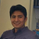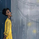# Squeeze Momentum Indicator [LazyBear]

366396 lượt xem
366396

Fixed a typo in the code where BB multiplier was stuck at 1.5. Thanks @ucsgears for bringing it to my notice.

Updated source: http://pastebin.com/UCpcX8d7

Use the updated source instead of the what TV shows below.

This is a derivative of John Carter's "TTM Squeeze" volatility indicator, as discussed in his book "Mastering the Trade" (chapter 11).

Black crosses on the midline show that the market just entered a squeeze ( Bollinger Bands are with in Keltner Channel). This signifies low volatility , market preparing itself for an explosive move (up or down). Gray crosses signify "Squeeze release".

Mr.Carter suggests waiting till the first gray after a black cross, and taking a position in the direction of the momentum (for ex., if momentum value is above zero, go long). Exit the position when the momentum changes (increase or decrease --- signified by a color change). My (limited) experience with this shows, an additional indicator like ADX / WaveTrend, is needed to not miss good entry points. Also, Mr.Carter uses simple momentum indicator , while I have used a different method (linreg based) to plot the histogram.

- Book: Mastering The Trade by John F Carter

List of all my indicators:

```//
// @author LazyBear
// List of all my indicators: https://www.tradingview.com/v/4IneGo8h/
//
study(shorttitle = "SQZMOM_LB", title="Squeeze Momentum Indicator [LazyBear]", overlay=false)

length = input(20, title="BB Length")
mult = input(2.0,title="BB MultFactor")
lengthKC=input(20, title="KC Length")
multKC = input(1.5, title="KC MultFactor")

useTrueRange = input(true, title="Use TrueRange (KC)", type=bool)

// Calculate BB
source = close
basis = sma(source, length)
dev = multKC * stdev(source, length)
upperBB = basis + dev
lowerBB = basis - dev

// Calculate KC
ma = sma(source, lengthKC)
range = useTrueRange ? tr : (high - low)
rangema = sma(range, lengthKC)
upperKC = ma + rangema * multKC
lowerKC = ma - rangema * multKC

sqzOn  = (lowerBB > lowerKC) and (upperBB < upperKC)
sqzOff = (lowerBB < lowerKC) and (upperBB > upperKC)
noSqz  = (sqzOn == false) and (sqzOff == false)

val = linreg(source  -  avg(avg(highest(high, lengthKC), lowest(low, lengthKC)),sma(close,lengthKC)),
lengthKC,0)

bcolor = iff( val > 0,
iff( val > nz(val), lime, green),
iff( val < nz(val), red, maroon))
scolor = noSqz ? blue : sqzOn ? black : gray
plot(val, color=bcolor, style=histogram, linewidth=4)
plot(0, color=scolor, style=cross, linewidth=2)
```¿Puedo tener acceso a esto por favor! Gracias !
Phản hồiThis is awesome. Anyone able to convert this into a buy and sell signal directly on the chart?
Phản hồi